UIHistories Project: A History of the University of Illinois by Kalev Leetaru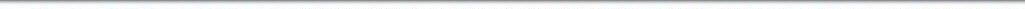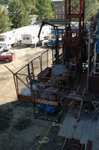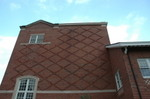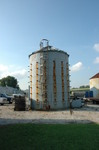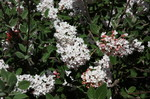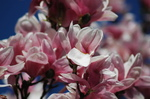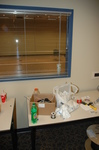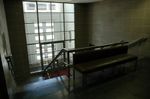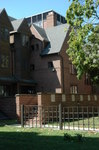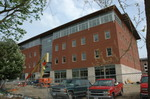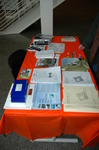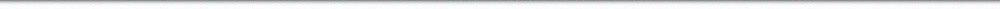N A V I G A T I O N D I G I T A L L I B R A R Y

## Repository: UIHistories Project: Board of Trustees Minutes - 1896 [PAGE 213]

Caption: Board of Trustees Minutes - 1896
This is a reduced-resolution page image for fast online browsing.

< Previous Page [Displaying Page 213 of 371] Next Page >
[VIEW ALL PAGE THUMBNAILS]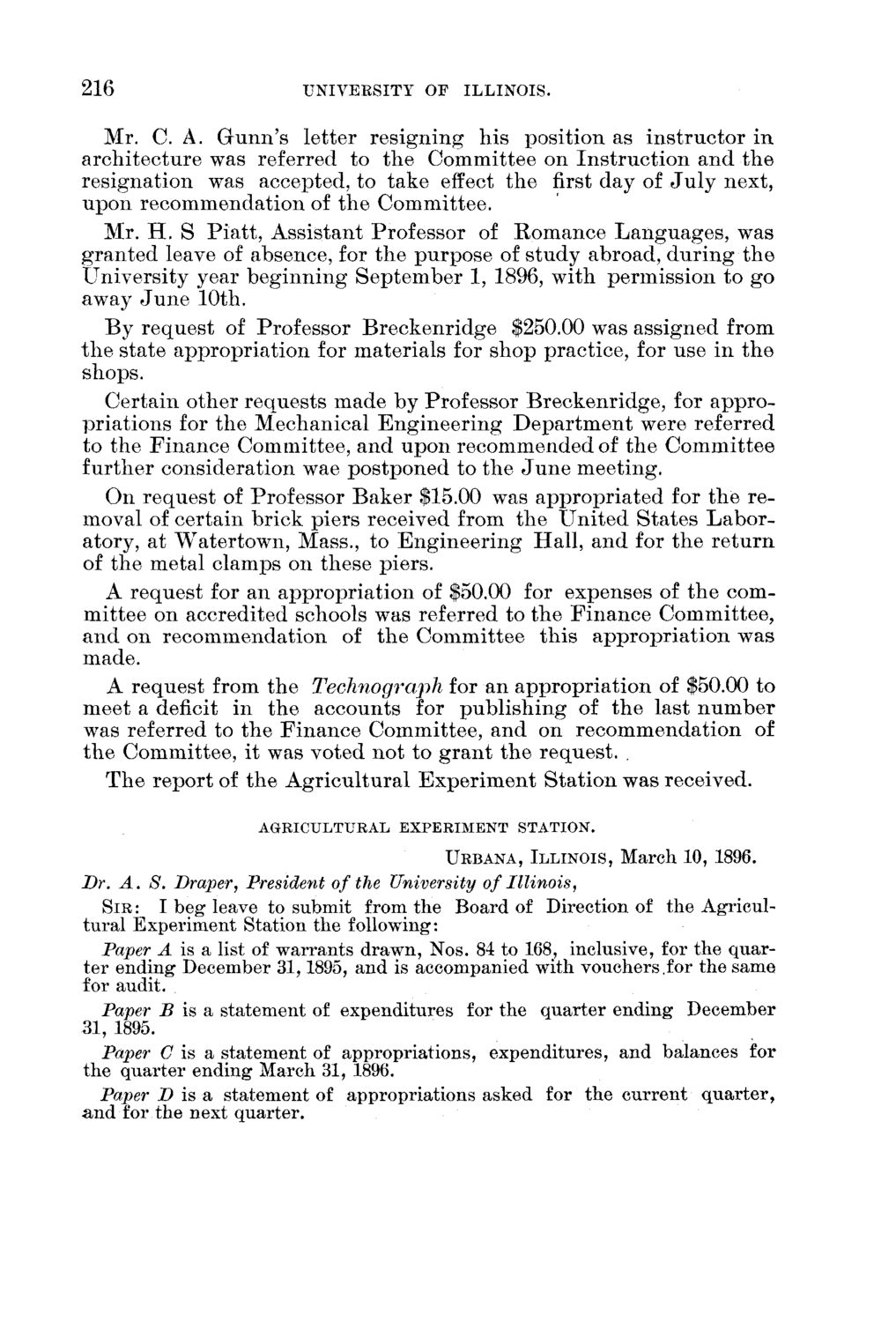### EXTRACTED TEXT FROM PAGE:

216

UNIVEESITY OF ILLINOIS.

Mr. C. A. Grunn's letter r e s i g n i n g h i s position as i n s t r u c t o r i n a r c h i t e c t u r e was referred to t h e C o m m i t t e e on I n s t r u c t i o n a n d t h e r e s i g n a t i o n was accepted, to take effect t h e first d a y of J u l y next, u p o n r e c o m m e n d a t i o n of t h e C o m m i t t e e . Mr. H . S P i a t t , A s s i s t a n t Professor of R o m a n c e L a n g u a g e s , was g r a n t e d leave of absence, for t h e p u r p o s e of s t u d y abroad, d u r i n g t h e U n i v e r s i t y year b e g i n n i n g S e p t e m b e r 1, 1896, with p e r m i s s i o n to g o away J u n e 10th. B y r e q u e s t of Professor B r e c k e n r i d g e \$250.00 was assigned from t h e state a p p r o p r i a t i o n for m a t e r i a l s for s h o p practice, for use in t h e shops. C e r t a i n o t h e r r e q u e s t s m a d e by Professor B r e c k e n r i d g e , for a p p r o p r i a t i o n s for t h e M e c h a n i c a l E n g i n e e r i n g D e p a r t m e n t were referred to t h e F i n a n c e Committee, a n d u p o n r e c o m m e n d e d of t h e C o m m i t t e e f u r t h e r consideration wae p o s t p o n e d to t h e J u n e m e e t i n g . O n r e q u e s t of Professor B a k e r \$15.00 was a p p r o p r i a t e d for t h e r e moval of certain brick piers received from t h e U n i t e d S t a t e s L a b o r atory, at W a t e r t o w n , Mass., to E n g i n e e r i n g H a l l , a n d for t h e r e t u r n of t h e metal clamps on these piers. A r e q u e s t for an a p p r o p r i a t i o n of \$50.00 for expenses of t h e comm i t t e e on accredited schools was referred to t h e F i n a n c e C o m m i t t e e , a n d on r e c o m m e n d a t i o n of t h e C o m m i t t e e t h i s a p p r o p r i a t i o n was made. A r e q u e s t from t h e Technograph for an a p p r o p r i a t i o n of \$50.00 to m e e t a deficit in t h e a c c o u n t s for p u b l i s h i n g of t h e last n u m b e r was referred to t h e F i n a n c e C o m m i t t e e , a n d on r e c o m m e n d a t i o n of t h e C o m m i t t e e , it was voted n o t to g r a n t t h e r e q u e s t . . T h e r e p o r t of t h e A g r i c u l t u r a l E x p e r i m e n t S t a t i o n was received.

AGRICULTURAL EXPERIMENT STATION.

URBANA, ILLINOIS, March 10, 1896.

Dr. A. S. Draper, President of the University of Illinois, SIR: I beg leave to submit from the Board of Direction of the Agricultural Experiment Station the following: Paper A is a list of warrants drawn, Nos. 84 to 168, inclusive, for the quarter ending December 31,1895, and is accompanied with vouchers.for the same for audit. Paper B is a statement of expenditures for the quarter ending December 31, 1895. Paper C is a statement of appropriations, expenditures, and balances for the quarter ending March 31, 1896. Paper D is a statement of appropriations asked for the current quarter, and for the next quarter.